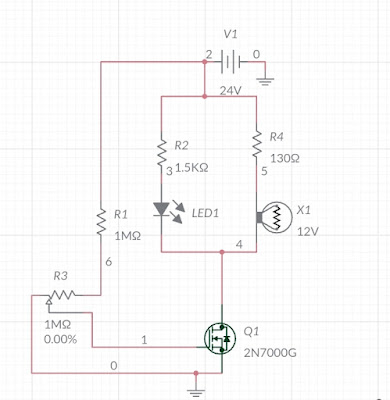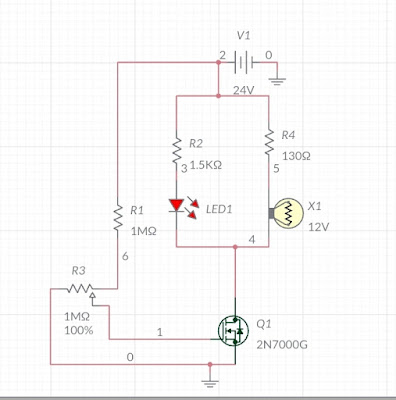In this circuit Light intensity is controlled by controlling drain to source current by controlling Gate to source voltage.MOSFET is a voltage controlled high frequency power switching device.

#### Components Required:-

1. MOSFET 2N7000G

2. Resistors

3. Lamp 12 Volt, 250 mA

4. LED

5. 24 Volts Battery

6. Potentiometer

#### Circuit Diagram:-Fig.1 Lamp intensity control circuit when Vgs=0Fig.2 Lamp intensity control circuit when Vgs=12V

#### Working:-

In the circuit a voltage divider is being used to limit Vgs upto 12 Volt and a current divider circuit is used to limit LED and Lamp current under 20 mA and 180 mA respectively. As the maximum current rating of MOSFET 2N7000G is 200 mA So R2 and R4 resistance value is being selected in such a way that overall resistance becomes 120 Ohm. This is why maximum current will flow less than 200 mA during On state of MOSFET.
Vgs = R3×V1/(R3+R1)

ILED  = 200 mA × R4/(R2+R4) = 15.95 mA

ILamp  = 200 mA×R2/(R2+R4) = 184.05 mA

IMOSFET = V1/R2 parallel R4 = 24/119.63 =  200.6 mA

#### Uses of Circuit :-

This circuit can be used to control any equipment which Working is directly dependent on current like Lamp, DC motors etc.

Author:- Amarjeet Singh Jamwal authored articles on Basic Electronics EngineeringElectric Traction, and Electronics Practical for INFO4EEE Website